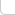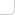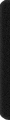# 某岛

… : "…アッカリ～ン . .. . " .. .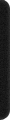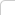February 21, 2013

## 培养皿问题

### Analysis:

A数列是每一行最左边元素的列坐标组成的数组，B则是最右边元素的列坐标数组。为了保证每一列也是连续的一段，A数组必须是一个先非递增，再非递减的数组，而B数组也类似。根据这一点，一个唯一的凸物对应一对唯一的A和B数组。因此我们就可以来考虑dp解法来选取A和B数组了。dp[i][j][k][b1][b2]表示最后一行为第i行的，第i行选取第j到第k列的方法数（b1和b2用来表示A序列和B数列分别是否已经在非递减/非递增状态）。对于第i行，一种情况是整个凸物只有第i行的元素，这个是很naive的情况；另外一种是除了第i行，还有前i-1行的一些元素，这样我们就可以利用到dp[i-1][?][?][?][?]的值了。具体状态转移要根据后两维的不同来分别处理。实际上，在求dp[i]时，我们需要求的都是某一段dp[i-1][j1 to j2][k1 to k2][?][?]所有元素的和，这是一个很经典的二维数组求子矩阵和问题，可以O(n^2)处理，之后O(1)计算。状态转移的时候还需要特别注意到，相等序列即符合非递增又符合非递减，所以A和B数组出现转折点的情况一定是出现了一个严格递增/严格递减的状态，这样可以保证每个状态被唯一表示，避免了重复计算。

dp[0/1][0/1][l][r]: 表示左右的增减状态为 b1, b2 ，当前区间为 [l, r] 时的状态。。
。。状态的时候先从上一轮预处理二维部分和数组。。之后分四种情况讨论即可。。。
Petr 的代码通过调整转移方向。。可以避免这步操作。。很优越。。）。。

。。实现的时候写了一个 Int 整数类。。自带取模。。

//}/* .................................................................................................................................. */

const int N = 109;

struct Int{
int val;

operator int() const{return val;}

Int(int val = 0):val(val){
val %= MOD; if (val < 0) val += MOD;
}
inline Int& operator +=(const Int& rhs){
INC(val, rhs);
return *this;
}
inline Int operator +(const Int& rhs) const{
return sum(val, rhs.val);
}
inline Int operator -(const Int& rhs) const{
return dff(val, rhs);
}
};

Int F[N][N], S[N][N]; bool A[N][N];
int n, m;

Int SS(int b1, int b2, int x1, int x2, int y1, int y2){
return S[b1][b2][x2+1][y2+1] - S[b1][b2][x2+1][y1] - S[b1][b2][x1][y2+1] + S[b1][b2][x1][y1];
}

public:
int count(vector <string> T) {

n = SZ(T), m = SZ(T); REP_2(i, j, n, m){
int t = isdigit(T[i][j]) ? T[i][j] - '0' : T[i][j] - 'a' + 10;
A[2*i][2*j] = t & 1, A[2*i][2*j+1] = t & 2;
A[2*i+1][2*j] = t & 4, A[2*i+1][2*j+1] = t & 8;
}

n <<= 1, m <<= 1; RST(F); Int res; REP(i, n){

RST(S); REP_4(b1, b2, l, r, 2, 2, m, m) S[b1][b2][l+1][r+1] = S[b1][b2][l][r+1] + S[b1][b2][l+1][r] - S[b1][b2][l][r] + F[b1][b2][l][r];

RST(F); REP(l, m) FOR(r, l, m){

if (A[i][r]) break;

F[l][r] = SS(0, 0, l, r, l, r) + Int(1);
F[l][r] = SS(0, 0, l, r, r+1, m-1) + SS(0, 1, l, r, r, m-1);
F[l][r] = SS(0, 0, 0, l-1, l, r) + SS(1, 0, 0, l, l, r);
F[l][r] = SS(0, 0, 0, l-1, r+1, m-1) + SS(0, 1, 0, l-1, r, m-1) + SS(1, 0, 0, l, r+1, m-1) + SS(1, 1, 0, l, r, m-1);

REP_2(b1, b2, 2, 2) res += F[b1][b2][l][r];
}
}

return res;
}
};


### 补充:

Codeforces Round #167 DIV 1 D. Dima and Figure

SGU 167. I-country.